# Common Core: High School - Geometry : Prove Geometric Theorems: Lines and Angles

## Example Questions

← Previous 1

### Example Question #1 : Prove Geometric Theorems: Lines And Angles

True or False: The Vertical Angle Theorem states that if two angles are vertical angles, then they have equal measure.

True

False

True

Explanation:

This is a true statement.  If two lines are intersecting, then their vertical angles are congruent.  Vertical angles are the opposite angles formed when two lines intersect.  The figure below shows an example of this.### Example Question #2 : Prove Geometric Theorems: Lines And Angles

Which of the following is the Same Side Interior Angles Theorem?

If a transversal intersects two parallel lines, then the interior angles on the same side of the transversal are supplementary

If a transversal intersects two parallel lines, then the exterior angles on the same side of the transversal are supplementary

If a transversal intersects two perpendicular lines, then the interior angles on the same side of the transversal are supplementary

If a transversal intersects two parallel lines, then the interior angles on the same side of the transversal are complementary

If a transversal intersects two parallel lines, then the interior angles on the same side of the transversal are supplementary

Explanation:

Consider the figure below.  If a transversal line intersects two parallel lines, then the two interior angles on the same side of the transversal (the two consecutive, interior angles of the transversal) are supplementary.  This means that they add up to be 180.### Example Question #1 : Prove Geometric Theorems: Lines And Angles

True or False: The Corresponding Angles Theorem states that if two parallel lines are cut by a transversal then the pair of corresponding angles are supplementary.

False

True

False

Explanation:

The Corresponding Angles Theorem states that if two parallel lines are cut by a transversal line then the pair of corresponding angles are congruent.  Corresponding angles are angles formed when a transversal line cuts two lines and they lie in the same position at each intersection.  The figure below illustrates corresponding lines.### Example Question #1 : Prove Geometric Theorems: Lines And Angles

Which of the following correctly state the Alternate Interior Angles Theorem?

If two parallel lines are cut by a transversal line, the corresponding interior angles are congruent

If two parallel lines are cut by a transversal line, the alternate interior angles are complementary

If two parallel lines are cut by a transversal line, the alternate interior angles are congruent

If two parallel lines are cut by a transversal line, the alternate interior angles are supplementary

If two parallel lines are cut by a transversal line, the alternate interior angles are congruent

Explanation:

The correct answer is "If two parallel lines are cut by a transversal line, the alternate interior angles are congruent."

Alternate interior angles are pairs of angles on the inner sides of parallel lines but on the opposite sides of a transversal line which is intersecting parallel lines.  These angles are always congruent.  Alternate interior angles are illustrated in the figure below.### Example Question #1 : Prove Geometric Theorems: Lines And Angles

True or False: Alternate exterior angles are congruent.

True

False

True

Explanation:

This is true according to the Alternate Exterior Angle Theorem which can be proven in a similar way to the Alternate Interior Angle Theorem.  Alternate exterior angles are illustrated in the figure below.### Example Question #232 : Congruence

What does it mean for two angles to be complementary angles?

Complementary angles are any two angles that sum to be 90

Complementary angles are any two angles in a triangle that sum to be 90

Complementary angles are any two angles that sum to be 180

Complementary angles are any two angles in a triangle that sum to be 180

Complementary angles are any two angles that sum to be 90

Explanation:

The definition of complementary angles is: any two angles that sum to 90.  We most often see these angles as the two angles in a right triangle that are not the right angle.  These two angles do not have to only be in right triangles, however.  Complementary triangles are any pair of angles that add up to be 90.

### Example Question #236 : Congruence

Lines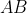and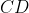are parallel.  Using this information, find the values for angles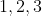, and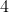.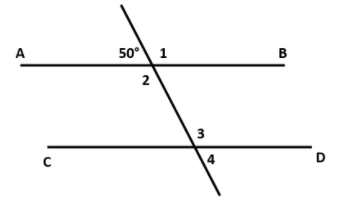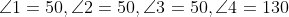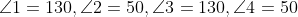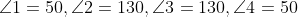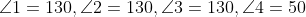Explanation:

We must use the fact that linesandare parallel lines to solve for the missing angles.  We will break it down to solve for each angle one at a time.

Angle:

We know that angle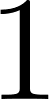’s supplementary angle.  Supplementary angles are two angles that add up to 180 degrees.  These two are supplementary angles because they form a straight line and straight lines are always 180 degrees.  So to solve for anglewe simply subtract it’s supplementary angle from 180.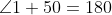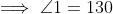Angle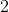:

We now know that angleis 130 degrees.  We can either use that fact that anglesandare opposite vertical angles to find the value of angleor we can use that fact that angle’s supplementary angle is the given angle of 50 degrees.  If we use the latter, we would use the same procedure as last time to solve for angle.  If we use the fact that anglesandare opposite vertical angles, we know that they are congruent.  Since angle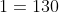then angle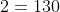.

Angle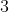:

To find anglewe can use the fact that anglesandare corresponding angles and therefore are congruent or we can use the fact that anglesandare alternate interior angles and therefore are congruent.  Either method that we use will show that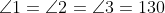.

Angle:

To find anglewe can use that fact that the given angle of 50 degrees and angleare alternate exterior angles and therefore are congruent, or we can use the fact that angleis angle's supplementary angle.  We know that the given angle and angleare alternate exterior angles so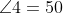.

### Example Question #1 : Prove Geometric Theorems: Lines And Angles

True or False: Lines AB and CD are parallel.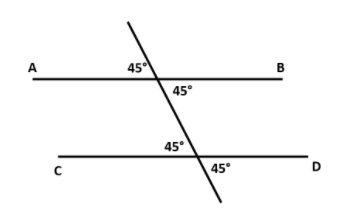True

False

True

Explanation:

We know that lines AB and CD are parallel due to the information we get from the angles formed by the transversal line.  There are:

• two pairs of congruent vertically opposite angles
• two pairs of congruent alternate interior angles
• two pairs of congruent alternate exterior angles
• two pairs of congruent corresponding angles

Just using any one of these facts is enough proof that lines AB and CD are parallel.

### Example Question #2 : Prove Geometric Theorems: Lines And Angles

Solve for angle 1.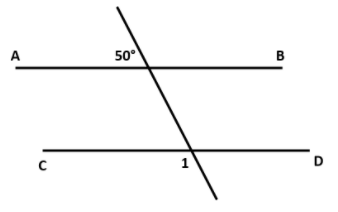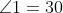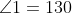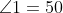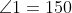Explanation:

Even though it may not be obvious at first, the given angle is actually a supplementary angle to angle.  This is because the given angle is corresponding (and therefore congruent) angles to the angle adjacent to angle.  Since they are supplementary we can set up the following equation.### Example Question #8 : Prove Geometric Theorems: Lines And Angles

Which of the following describes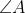and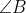?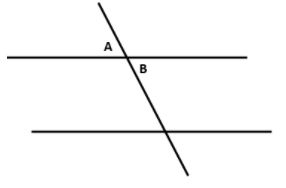These are vertically opposite angles, there is not enough information to determine any further relation

These are corresponding angles, there is not enough information to determine any further relation

These are vertically opposite angles, therefore they are equal

These are corresponding angles, therefore they are equal